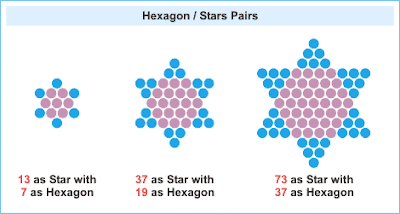## Friday, 7 June 2019

### Star NumbersFigure 1: Chinese Checker Board with 121 holes

To quote from Wikipedia:

A star number is a centered figurate number, a centered hexagram (six-pointed star), such as the one that Chinese checkers is played on. The nth star number is given by the formula $S_n = 6*n*(n − 1) + 1$. The first 43 star numbers are:
1, 13, 37, 73, 121, 181, 253, 337, 433, 541, 661, 793, 937, 1093, 1261, 1441, 1633, 1837, 2053, 2281, 2521, 2773, 3037, 3313, 3601, 3901, 4213, 4537, 4873, 5221, 5581, 5953, 6337, 6733, 7141, 7561, 7993, 8437, 8893, 9361, 9841, 10333, 10837 (sequence A003154 in the OEIS).
The digital root of a star number is always 1 or 4, and progresses in the sequence 1, 4, 1. The last two digits of a star number in base 10 are always 01, 13, 21, 33, 37, 41, 53, 61, 73, 81, or 93.

Unique among the star numbers is 35113, since its prime factors (i.e. 13, 37 and 73) are also consecutive star numbers.

Geometrically, the n-th star number is made up of a central point and 12 copies of the (n−1)-th triangular number — making it numerically equal to the n-th centered dodecagonal number, but differently arranged.

Infinitely many star numbers are also triangular numbers, the first four being:

• $S_1 = 1 = T_1$
• $S_7 = 253 = T_{22}$
• $S_{91} = 49141 = T_{313}$
• $S_{1261} = 9533161 = T_{4366}$

Sequence A156712 in the OEIS: star numbers that are also triangular numbers.

Infinitely many star numbers are also square numbers, the first four being:

• $S_1 = 1^2$
• $S_5 = 121 = 11^2$
• $S_{45} = 11881 = 109^2$
• $S_{441} = 1164241 = 1079^2$

Sequence A054318 in the OEIS: star numbers that are also square numbers.

A star prime is a star number that is prime. The first few star primes (sequence A083577 in the OEIS) are 13, 37, 73, 181, 337, 433, 541, 661, 937.

In terms of my numbered days, I have a star prime coming up in the not too distant future. It is 25741, the 33rd star prime. This is in another 109 days at the time of this post. While researching for information about star numbers, I came across this interesting site (hosted on Google Sites) titled Mathematical Monotheism created by Leo Tavales. There is a lot of information and analysis there involving Hebrew and Greek gematria. All very interesting. He's created a PDF file as well which I've downloaded and added to my Calibre library. I've included one of his diagrams in Figure 2:Figure 2: Star of Genesis, created by Leo Tavales

Leo's depicts the content on his site as BIBLICAL NUKES OVER THE FORTIFIED CITIES OF ATHEISM, SECULARISM AND MATERIALISM. He is a committed Christian but nonetheless his site has served to remind me of gematria which I became familiar with in my twenties but have not explored in any depth since then. The numbers 7, 13, 37 and 73 play a central role in Leo's Genesis analysis. Figure 3 shows shows the star-hexagon relationship between these numbers:Figure 3: geometrical relationship between 7 13, 37 and 73

I'll go into this in more detail in a later blog post dealing with gematria but suffice to say here that the words in the first line of the Bible, Genesis 1:1, add to 2701 in Hebrew and this number has some interesting properties:

The number 2701 is the sum of the first 73 triangular numbers. Notice that 37 and 73 are perfect mirror reflections of one another. These are the PRIME FACTORS of Genesis 1:1. In fact, the ONLY way to produce 2701 as a product of two numbers (besides 2701 × 1) is 37 × 73. Moreover, 2701 is the first and ONLY Composite number up to 10,000 that yields its PRIME FACTORS when it is added to its mirror reflection:

2701 + 1072 = 3773

The number 2701 happens to be the 37th Hexagonal number and the 73rd Triangular number (these are the ONLY two ways to represent 2701 counters as a geometric polygon)

The numbers 37 and 73 are also the first and ONLY mirrored Prime numbers, out of the first 100,000 that have been checked, whose PRIME ORDERS (12 and 21) are mirror reflections as well. In fact, we have the following:

37 = 12th Prime and 12^2 = 144

73 = 21st Prime and 21^2 = 441

Another source has this to say about the number 37:

The Number 37 appears in two Hexagon/Star pairs. It is the Star in the 19/37 pair and the Hexagon in the 37/73 pair. This is an extremely rare property. There are only twelve numbers less than seven thousand trillion that can be represented both as a Hexagon and a Star. The Number 37 is the only such number less than a thousand.